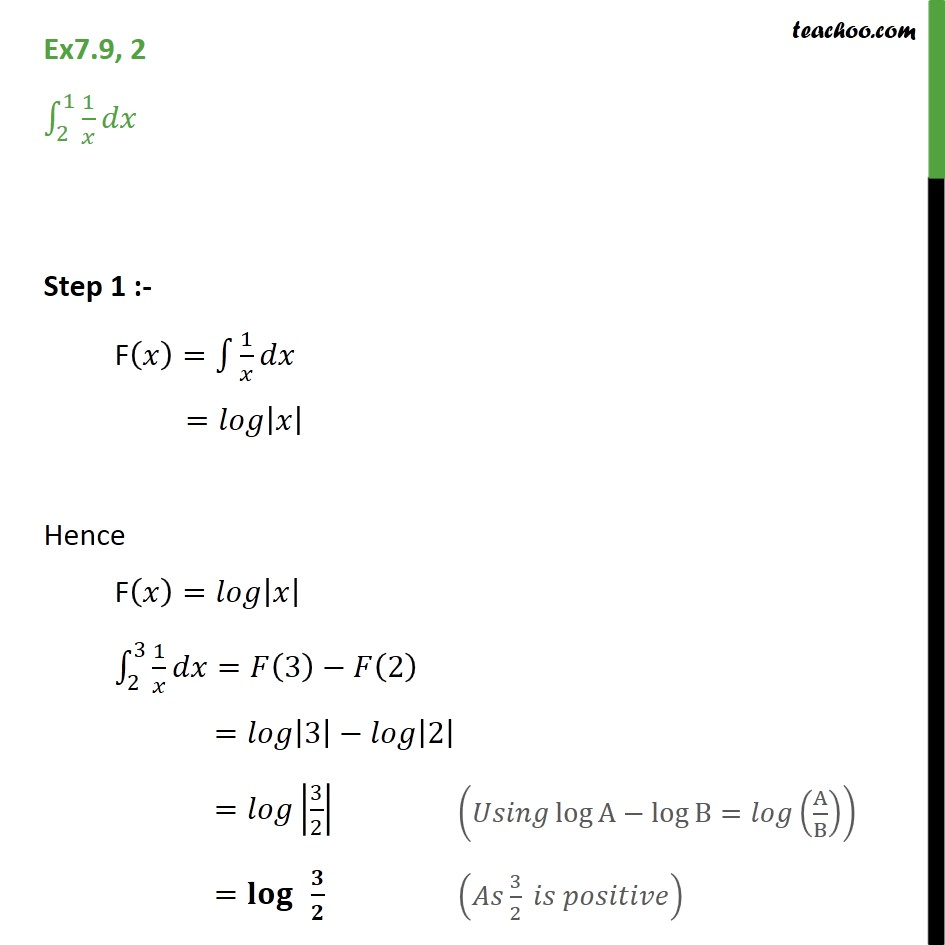1. Chapter 7 Class 12 Integrals
2. Concept wise
3. Definite Integration - By Formulae

Transcript

Ex 7.9, 2 - Chapter 7 Class 12 Integration - NCERT Solution Integrate ∫ 2 -> 1 1/x dx Step 1 :- F(x) = ∫ 1/x dx = log |x| Hence F(𝑥)=𝑙𝑜𝑔|𝑥| ∫1_2^3▒1/𝑥 𝑑𝑥=𝐹(3)−𝐹(2) =𝑙𝑜𝑔|3|−𝑙𝑜𝑔|2| =𝑙𝑜𝑔|3/2| (𝑈𝑠𝑖𝑛𝑔 log⁡A−log⁡B=𝑙𝑜𝑔(A/B)) =𝐥𝐨𝐠⁡ 𝟑/𝟐 (𝐴𝑠 3/2 𝑖𝑠 𝑝𝑜𝑠𝑖𝑡𝑖𝑣𝑒)

Definite Integration - By Formulae